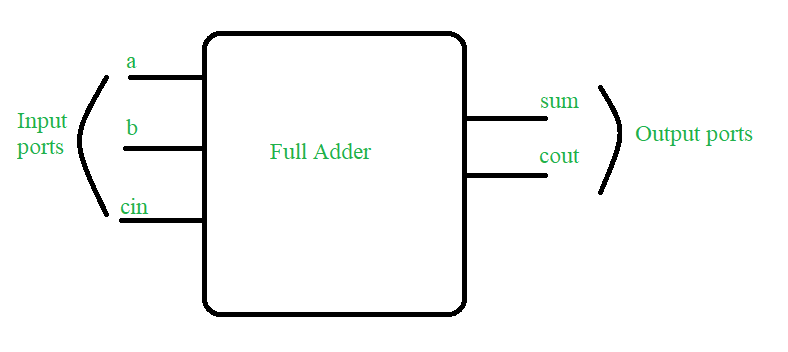# Full Adder using Verilog HDL

• Last Updated : 13 Sep, 2021

In this article, we will discuss the overview part of Full Adder using Verilog HDL. And the objective to understand the concept and will implement using Verilog HDL code for Full Adder. Let’s discuss it one by one.

Prerequisite Full Adder in Digital Logic

Attention reader! Don’t stop learning now. Get hold of all the important CS Theory concepts for SDE interviews with the CS Theory Course at a student-friendly price and become industry ready.

Problem Statement :
Write a Verilog HDL to design a Full Adder. Let’s discuss it step by step as follows.

Step-1 :
Concept –
Full Adder is a digital combinational Circuit which is having three input a, b and cin and two output sum and cout. Below Truth Table is drawn to show the functionality of the Full Adder.Figure shows the block diagram of design requirements : Full Adder

Step-2 :
Truth Table –

Step-3 :
Verilog HDL code for Full Adder (Design Part) –

```// Code your design : Full Adder
input a,b,cin;
output sum,cout;
wire x,y,z;

// instantiate building blocks of full adder
or o1(cout,y,z);

input a,b;
output s,c;

// gate level design of half adder
xor x1(s,a,b);
and a1(c,a,b);

Step-4 :
Test bench –

```// Code your testbench here
reg a,b,cin;
wire sum,cout;

// instantiate the DUT block

// this particular line is added to dump the file on online simulator
initial begin \$dumpfile("full_tb.vcd");\$dumpvars(); end

// insert all the inputs
initial begin a=1'b1;  #4; a=1'b0;#10 \$stop();end
initial begin b=1'b1; forever #2 b=~b;end
initial begin cin=1'b1;forever #1 cin=~cin; #10 \$stop();end

// monitor all the input and output ports at times
// when any of the input changes its state

initial begin \$monitor(" time=%0d A=%b B=%b
Cin=%b Sum=%b Cout=%b",\$time,a,b,cin,sum,cout);end

Step-5 :
Expected Output –

```time=0 A=1 B=1 Cin=1 Sum=1 Cout=1
time=1 A=1 B=1 Cin=0 Sum=0 Cout=1
time=2 A=1 B=0 Cin=1 Sum=0 Cout=1
time=3 A=1 B=0 Cin=0 Sum=1 Cout=0
time=4 A=0 B=1 Cin=1 Sum=0 Cout=1
time=5 A=0 B=1 Cin=0 Sum=1 Cout=0
time=6 A=0 B=0 Cin=1 Sum=1 Cout=0
time=7 A=0 B=0 Cin=0 Sum=0 Cout=0
time=8 A=0 B=1 Cin=1 Sum=0 Cout=1
time=9 A=0 B=1 Cin=0 Sum=1 Cout=0
time=10 A=0 B=0 Cin=1 Sum=1 Cout=0
time=11 A=0 B=0 Cin=0 Sum=0 Cout=0
time=12 A=0 B=1 Cin=1 Sum=0 Cout=1
time=13 A=0 B=1 Cin=0 Sum=1 Cout=0```
My Personal Notes arrow_drop_up